"3rd Grade Math Games - multiplication and division" App Analytics: ASO Keyword Monitoring | ASOTools

# "3rd Grade Math Games - multiplication and division" App Analytics: ASO Keyword Monitoring | ASOTools

Are you doing 3rd Grade Math Games - multiplication and division app analytics for better app store ASO strategies? Whether 3rd Grade Math Games - multiplication and division in app store keep on top? What keyword tactics 3rd Grade Math Games - multiplication and division take to rank well in app store? Are app store keywords like "multiplication and division games for 3rd graders", "division and multiplication math games homeschool", "3rd grade math games multiplication and division" matter in 3rd Grade Math Games - multiplication and division ranking?

Free ASO tools for Google Play & App Store, ASOTools will reveal the app store keywords secrets of 3rd Grade Math Games - multiplication and division.

## App Analytics: 3rd Grade Math Games - multiplication and division

3rd Grade Math Games - multiplication and division, published by Nuttapong Tongrungsri in app store, released on 2015-10-29, and last updated 2016-01-21. It belongs to all category, and all category is ranking no rank in app store. Currently, 3rd Grade Math Games - multiplication and division has 419 ratings, the average rating is 3. Additionally, it had - downloads and - revenue last month in app store worldwide, and the daily active users is -.

More 3rd Grade Math Games - multiplication and division info and other app analytics can be found freely in ASOTool.

## ASO Keyword Monitoring: 3rd Grade Math Games - multiplication and division

### 1.3rd Grade Math Games - multiplication and division Top 10 App Store Keywords

 Keyword Search Volume KD Related Apps 3rd Grade Math Games - multiplication and division's Rank multiplication and division games for 3rd graders - - 8 35 1 division and multiplication math games homeschool 7 - 5 6 1 3rd grade math games multiplication and division 8 - 11 62 1 math games 3 grade - - 42 220 1 3rd grade math games - multiplication and division 9 - 26 201 1 free math games for 3rd grade - - 23 226 1 3rd games - - 22 196 1 third grade math games 5 4605 26 196 1 free 3rd grade math games - - 22 227 1 math fundamentals - - 6 18 1

App store keywords matter for ASO. If your app is similar to 3rd Grade Math Games - multiplication and division, when you optimize app store keyword ranking, pay attention to the metrics above. ASOTools will show you all of them for free.

Obviously, 3rd Grade Math Games - multiplication and division is ranking for "multiplication and division games for 3rd graders", "division and multiplication math games homeschool", "3rd grade math games multiplication and division", "math games 3 grade", "3rd grade math games - multiplication and division", "free math games for 3rd grade", "3rd games", "third grade math games", "free 3rd grade math games", and "math fundamentals" these top 10 keywords in app store.

Also, you'll find 3rd Grade Math Games - multiplication and division's position in app store search result. 3rd Grade Math Games - multiplication and division is ranking 1 for multiplication and division games for 3rd graders, ranking 1 for division and multiplication math games homeschool, ranking 1 for 3rd grade math games multiplication and division, and ranking 1 for math games 3 grade. For more 3rd Grade Math Games - multiplication and division keyword monitoring, please visit asotools.io.

### 2. Top 5 Keyword-Related Apps& App Analytics

• multiplication and division games for 3rd graders  Related Apps & App Analytics
 Apps Average Star Ratings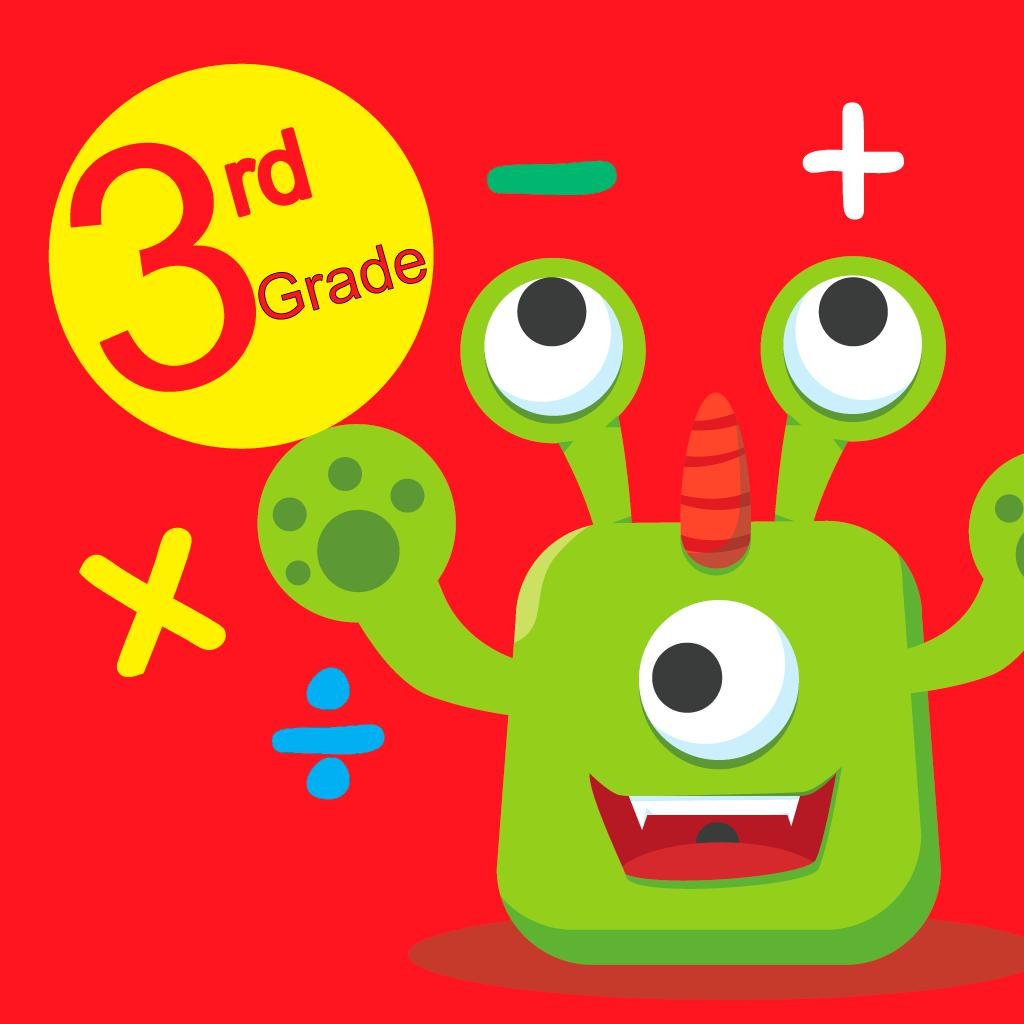3rd Grade Math Games - multiplication and division 3 419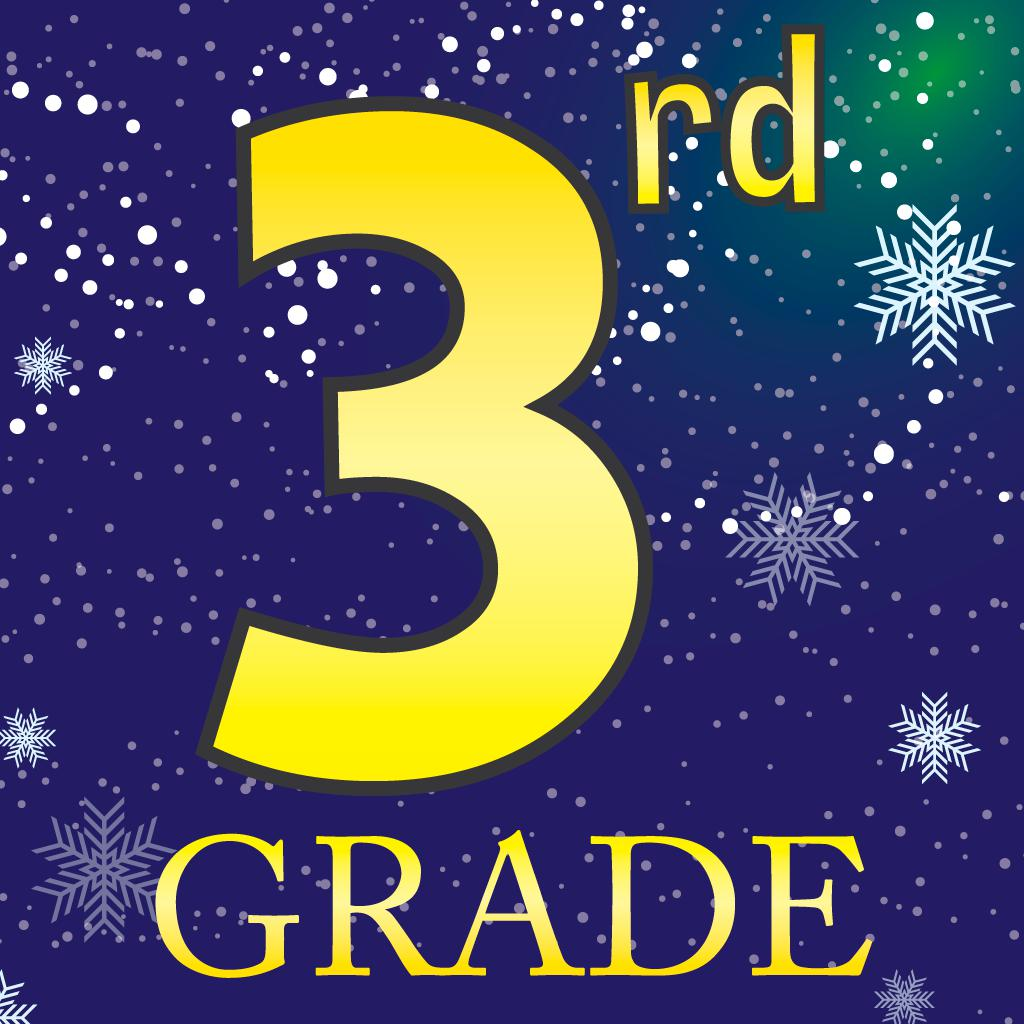3rd Grade Math multiplication and division learning for kids 2 5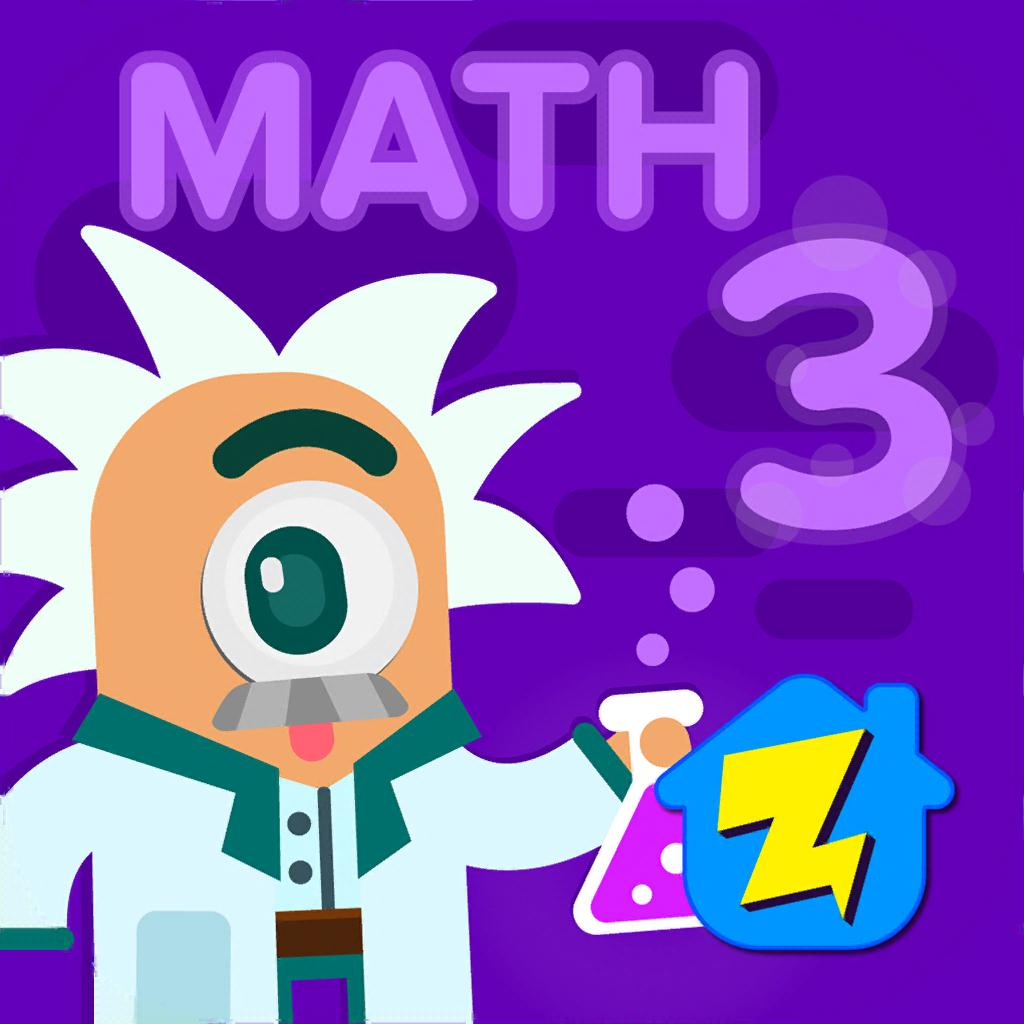3rd Grade Math: Fun Kids Games-Zapzapmath Home - Play & Learn 4 444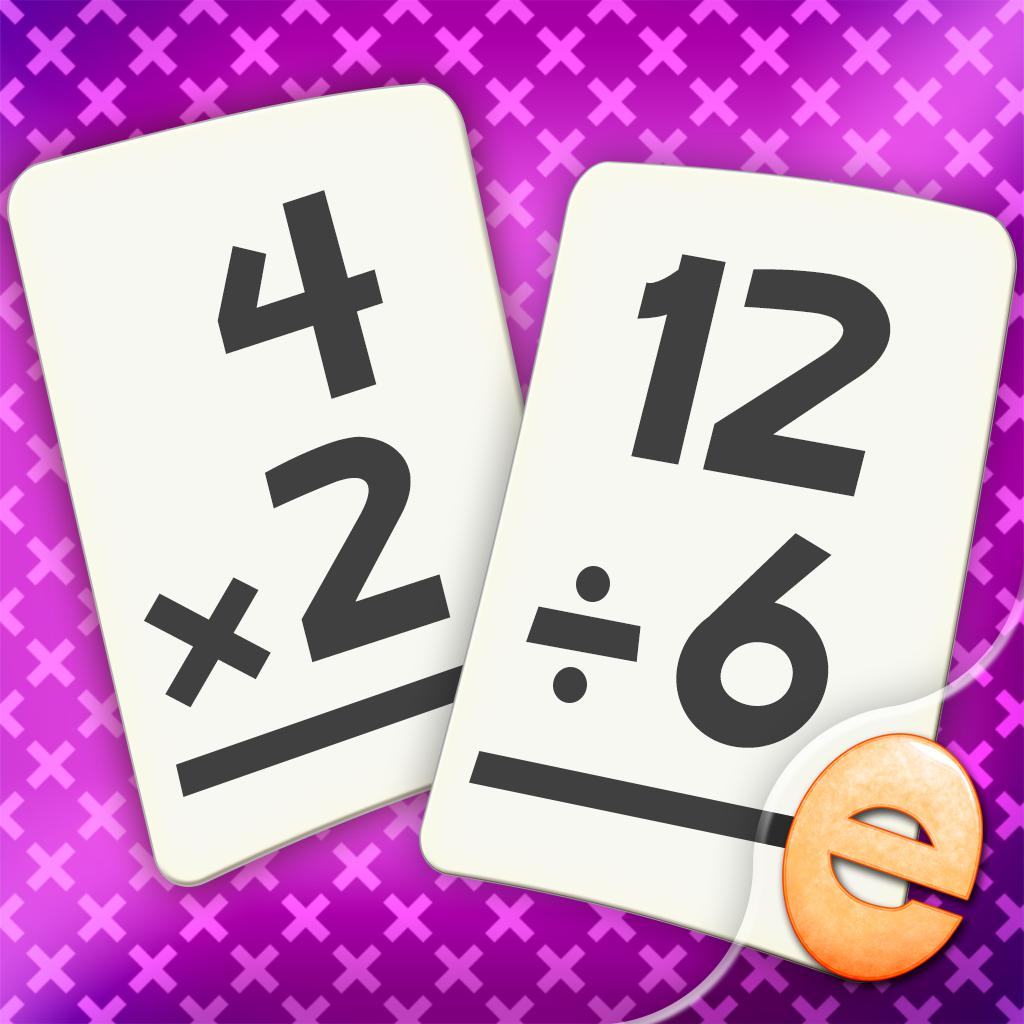Multiplication and Division Math Flashcard Match Games for Kids in 2nd and 3rd Grade 3 3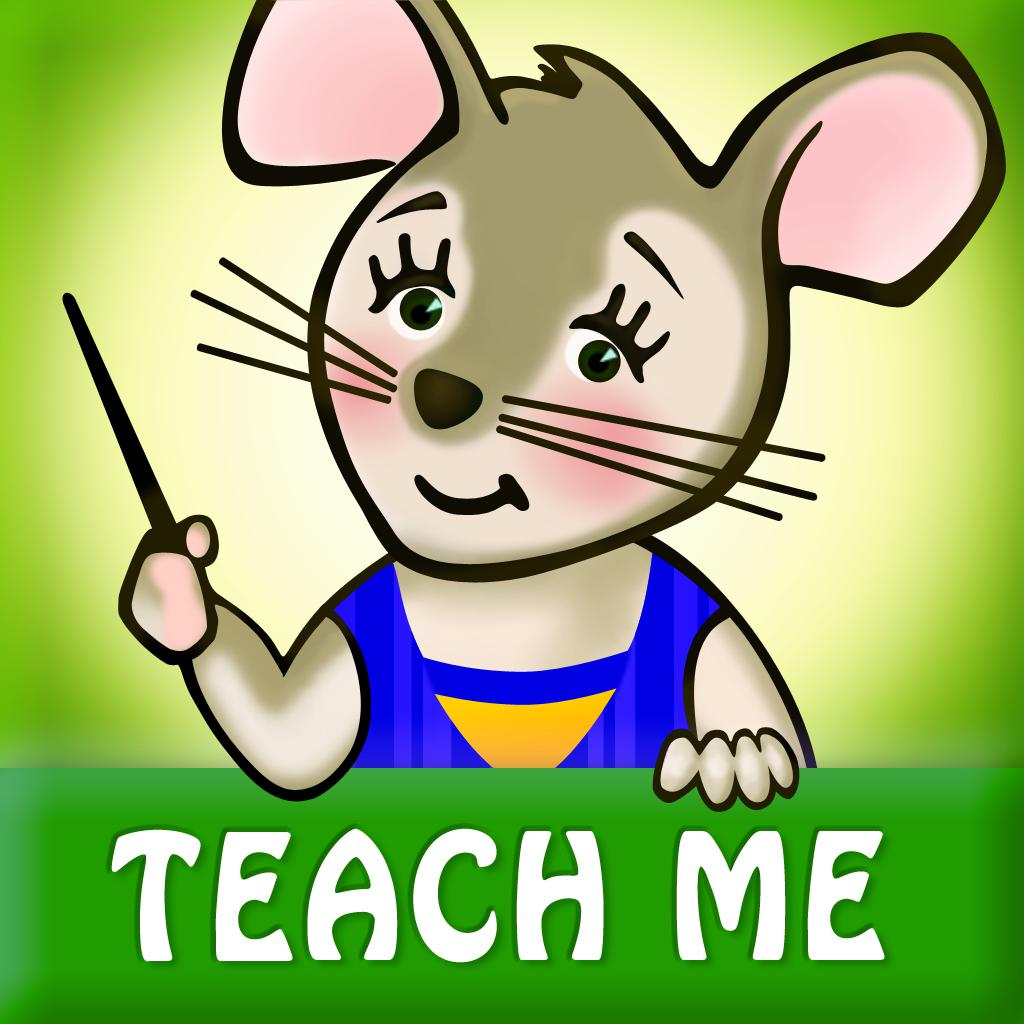TeachMe: 3rd Grade-Math, reading, & spelling 3 42

"multiplication and division games for 3rd graders" has 35 apps. Top 5 are 3rd Grade Math Games - multiplication and division, 3rd Grade Math multiplication and division learning for kids, 3rd Grade Math: Fun Kids Games, Multiplication and Division Math Flashcard Match Games for Kids in 2nd and 3rd Grade, and TeachMe: 3rd Grade.

• division and multiplication math games homeschool  Related Apps & App Analytics
 Apps Average Star Ratings3rd Grade Math Games - multiplication and division 3 419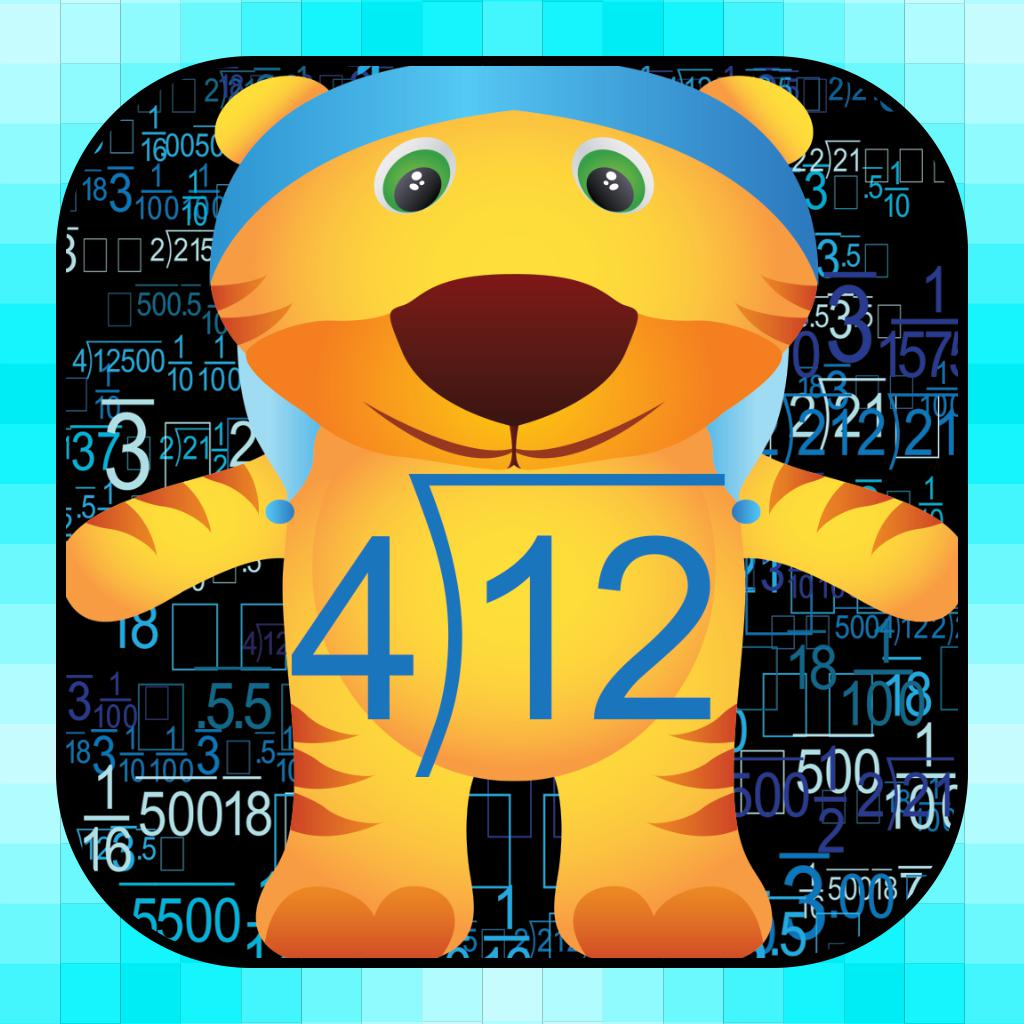Division and multiplication math games homeschool - -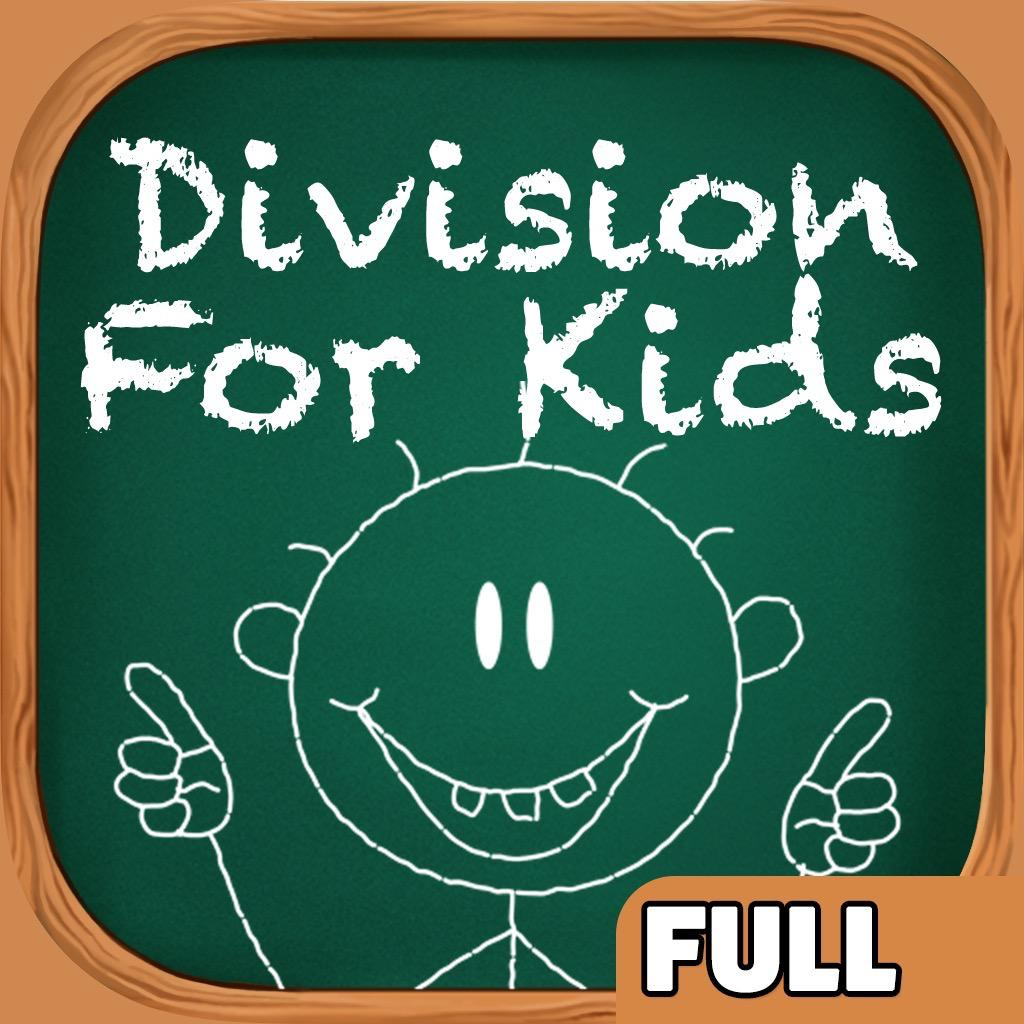Division Games for Kids - Full-Practice & Learn Basic Math 5 24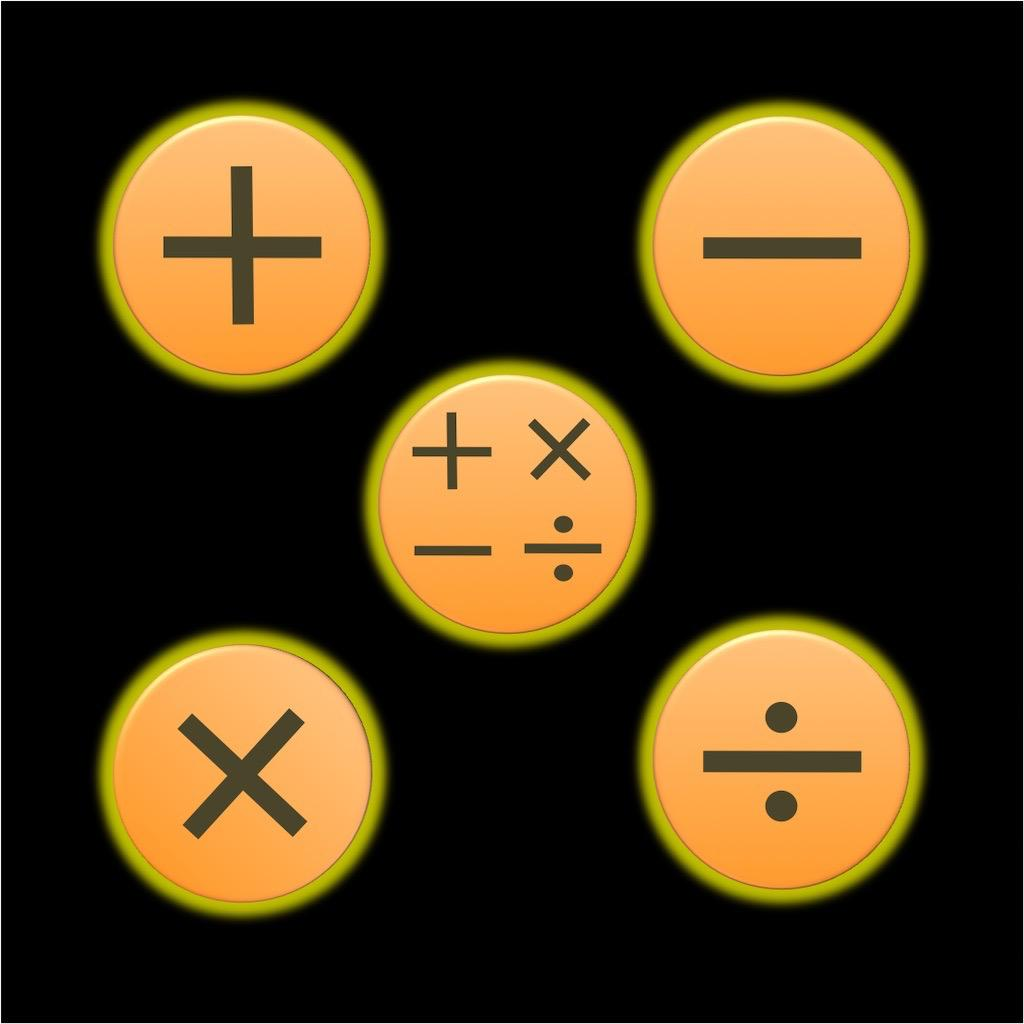Memory Math: +, -, x, /-improve memory & practice math - -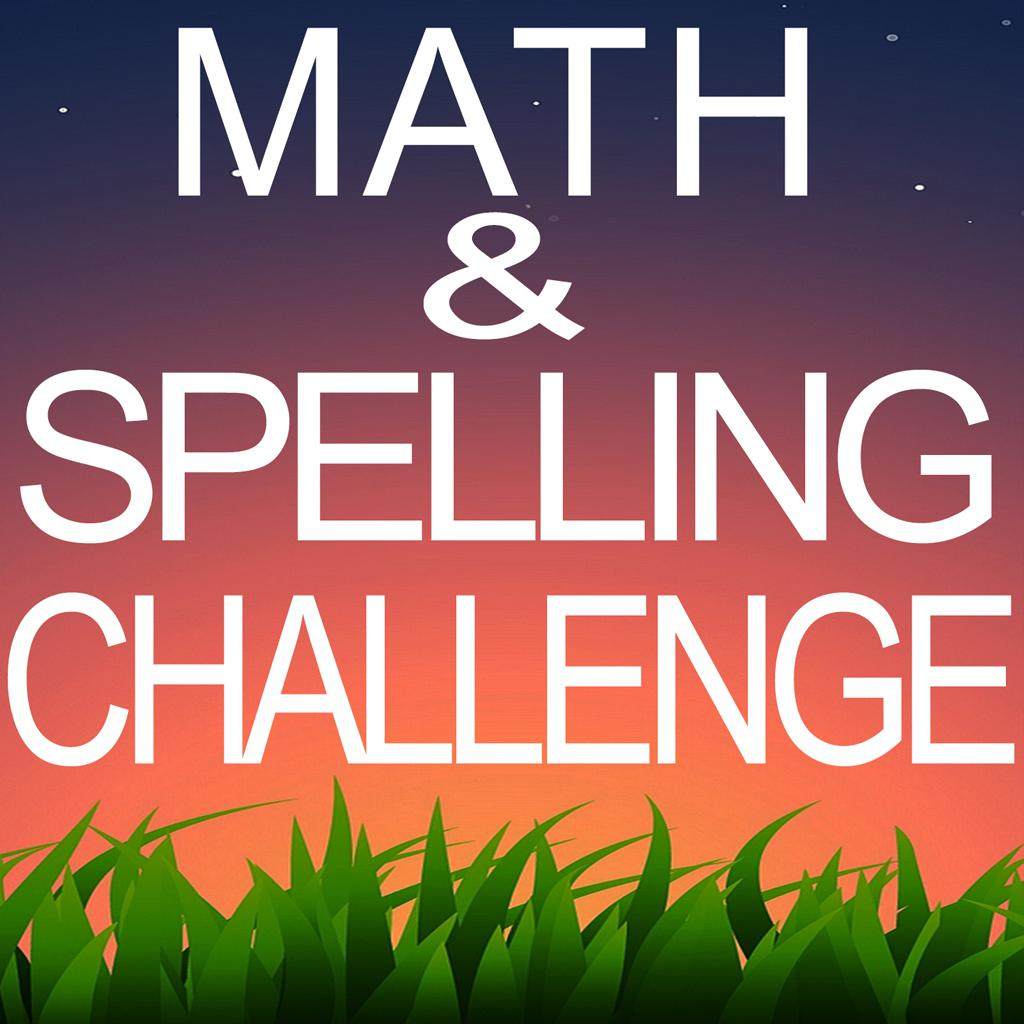Math and Spelling First Grade Challenge for all 1 1

"division and multiplication math games homeschool" has 6 related apps in total. Top 5 are 3rd Grade Math Games - multiplication and division, Division and multiplication math games homeschool, Division Games for Kids - Full, Memory Math: +, -, x, /, and Math and Spelling First Grade Challenge for all.

• 3rd grade math games multiplication and division  Related Apps & App Analytics
 Apps Average Star Ratings3rd Grade Math Games - multiplication and division 3 419Math Game Brain Trainer with Addition, Subtraction, Multiplication & Division, also one of the Best Free Learning Games for Kids, Adults, Middle School, 3rd, 4th, 5th, 6th and 7th Grade 3 709Prodigy: Kids Math Game-Math practice for grades 1-8 4 415473rd Grade Math multiplication and division learning for kids 2 5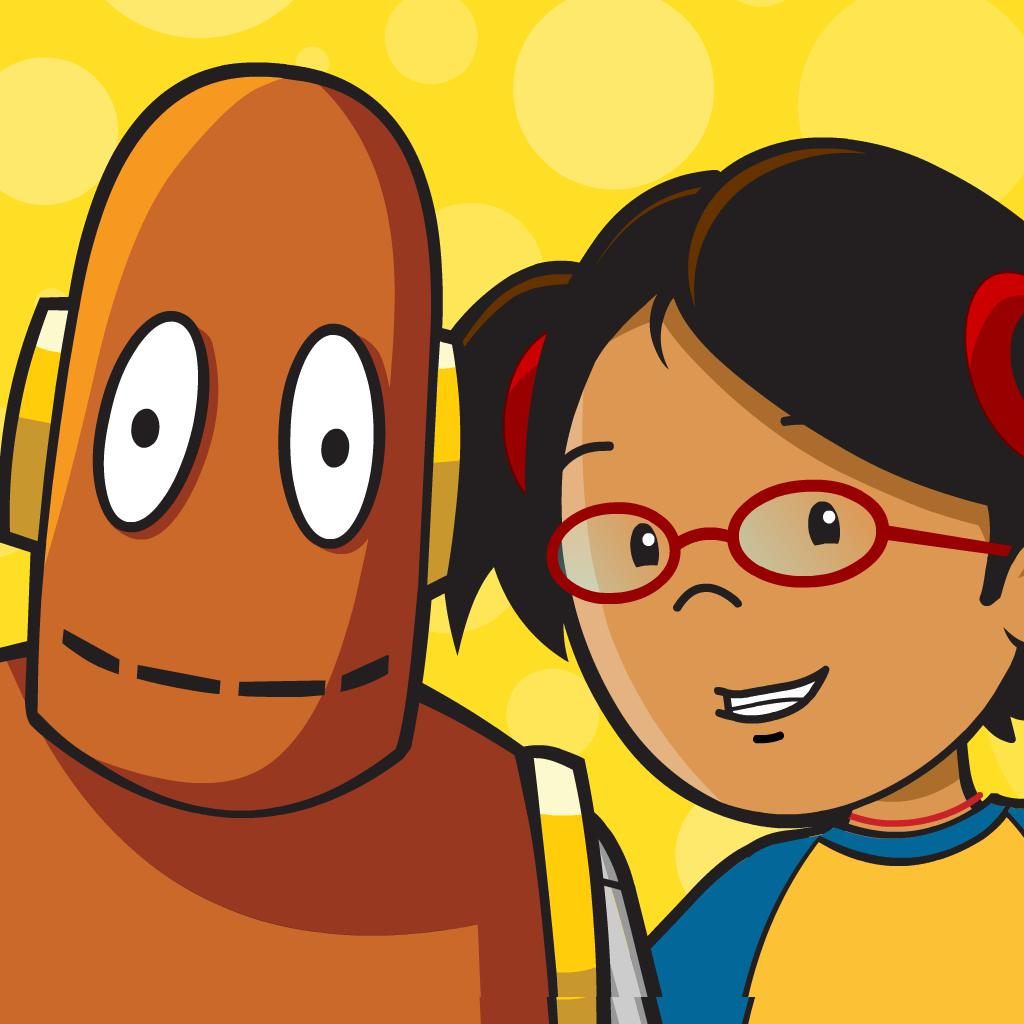BrainPOP Jr. Movie of the Week-Inspire K-3 learners 4 16211

"3rd grade math games multiplication and division" has 62 related apps in app store. Top 5 are 3rd Grade Math Games - multiplication and division, Math Game Brain Trainer with Addition, Subtraction, Multiplication & Division, also one of the Best Free Learning Games for Kids, Adults, Middle School, 3rd, 4th, 5th, 6th and 7th Grade, Prodigy: Kids Math Game, 3rd Grade Math multiplication and division learning for kids, and BrainPOP Jr. Movie of the Week.

• math games 3 grade  Related Apps & App Analytics
 Apps Average Star Ratings3rd Grade Math Games - multiplication and division 3 419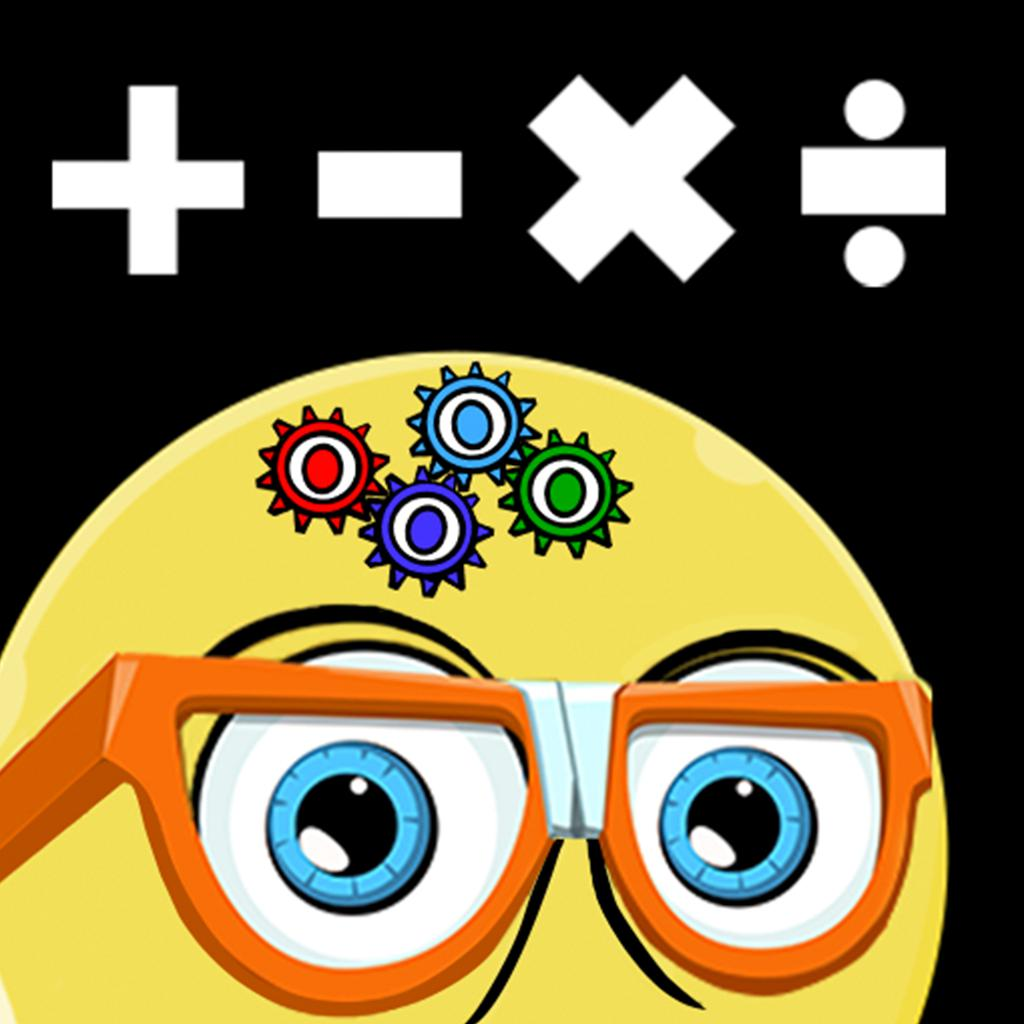Math Balance: Educational Game-Games For 6-10 Year Old Kids 4 1496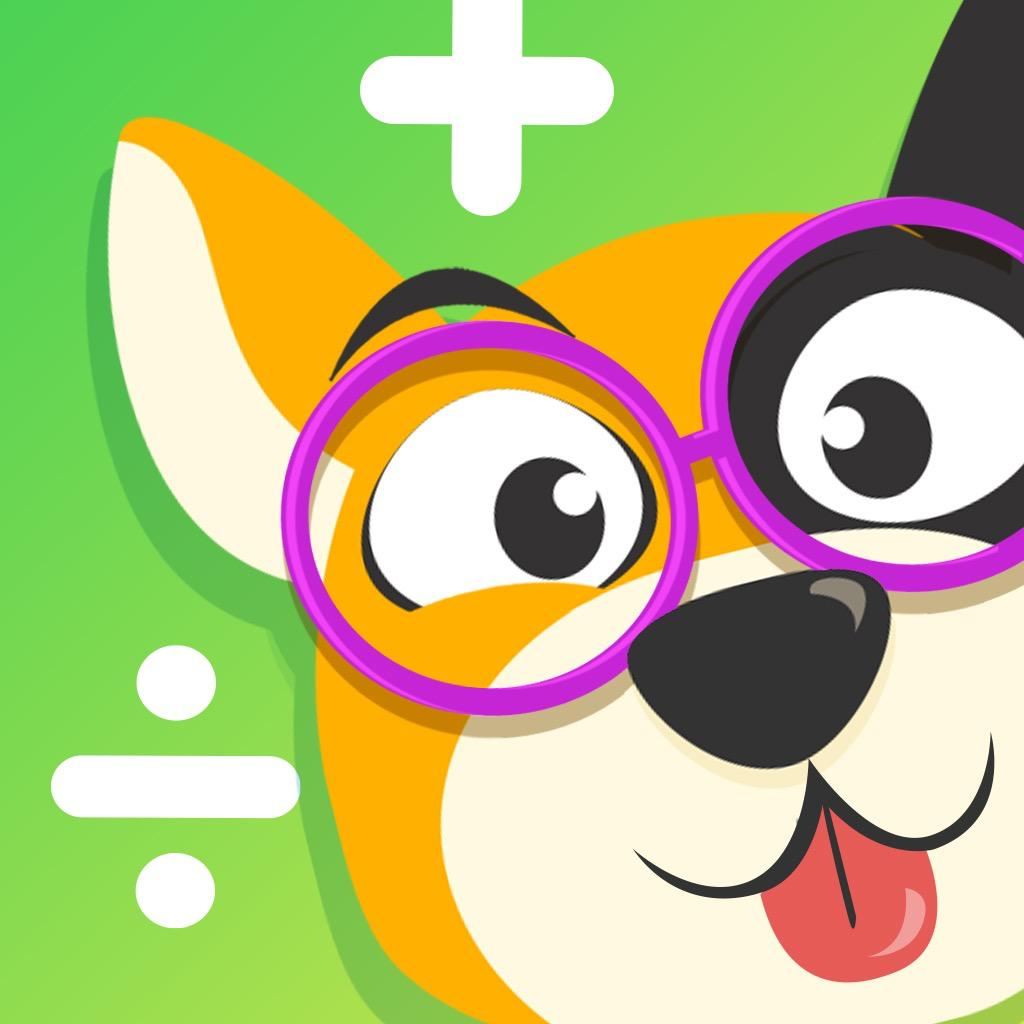Math Learner: Learning Game-Fun extra classroom problems 4 114570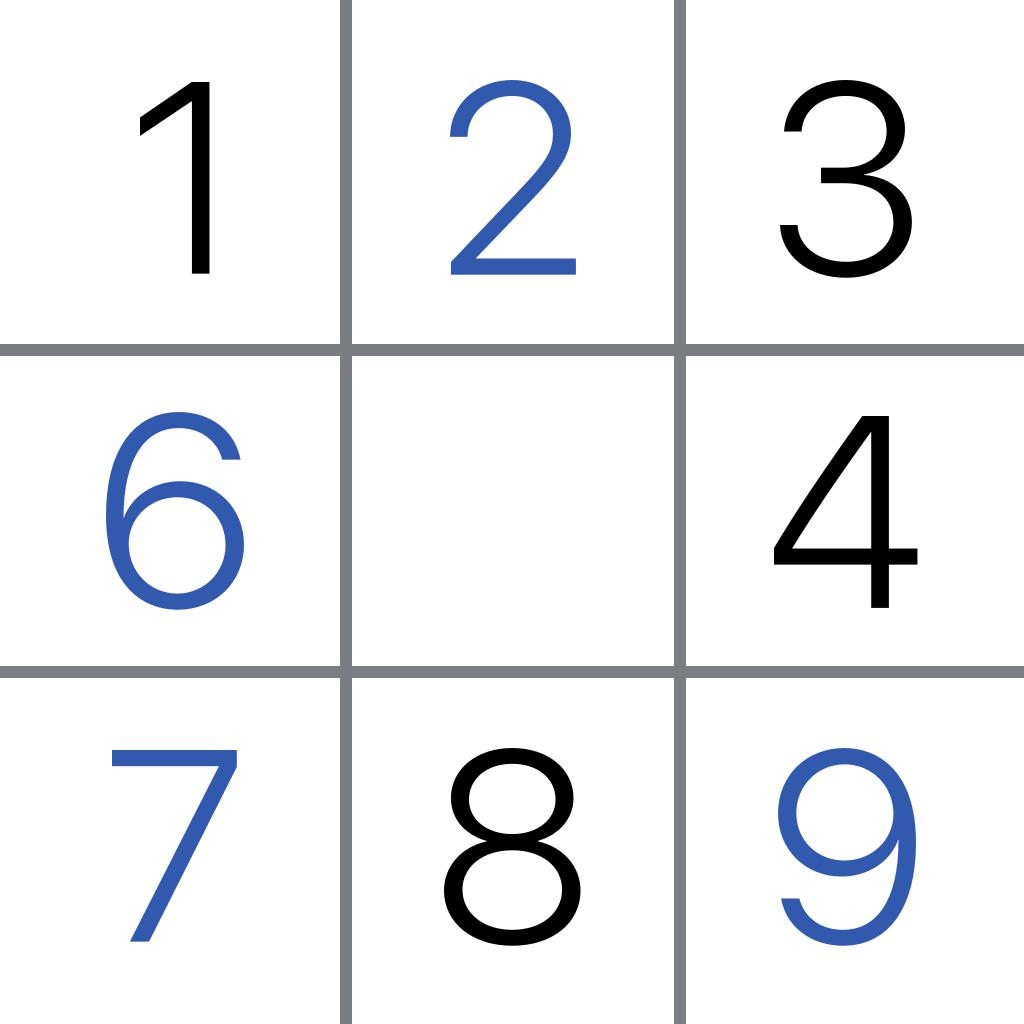Sudoku.com - Sudoku Puzzle-Logic puzzles and number games 4 1124431Quizlet-Flashcards & Study Tools 5 486330

"math games 3 grade" has 220 related apps. Top 5 apps are 3rd Grade Math Games - multiplication and division, Math Balance: Educational Game, Math Learner: Learning Game, Sudoku.com - Sudoku Puzzle, and Quizlet.

• 3rd grade math games - multiplication and division  Related Apps & App Analytics
 Apps Average Star Ratings3rd Grade Math Games - multiplication and division 3 419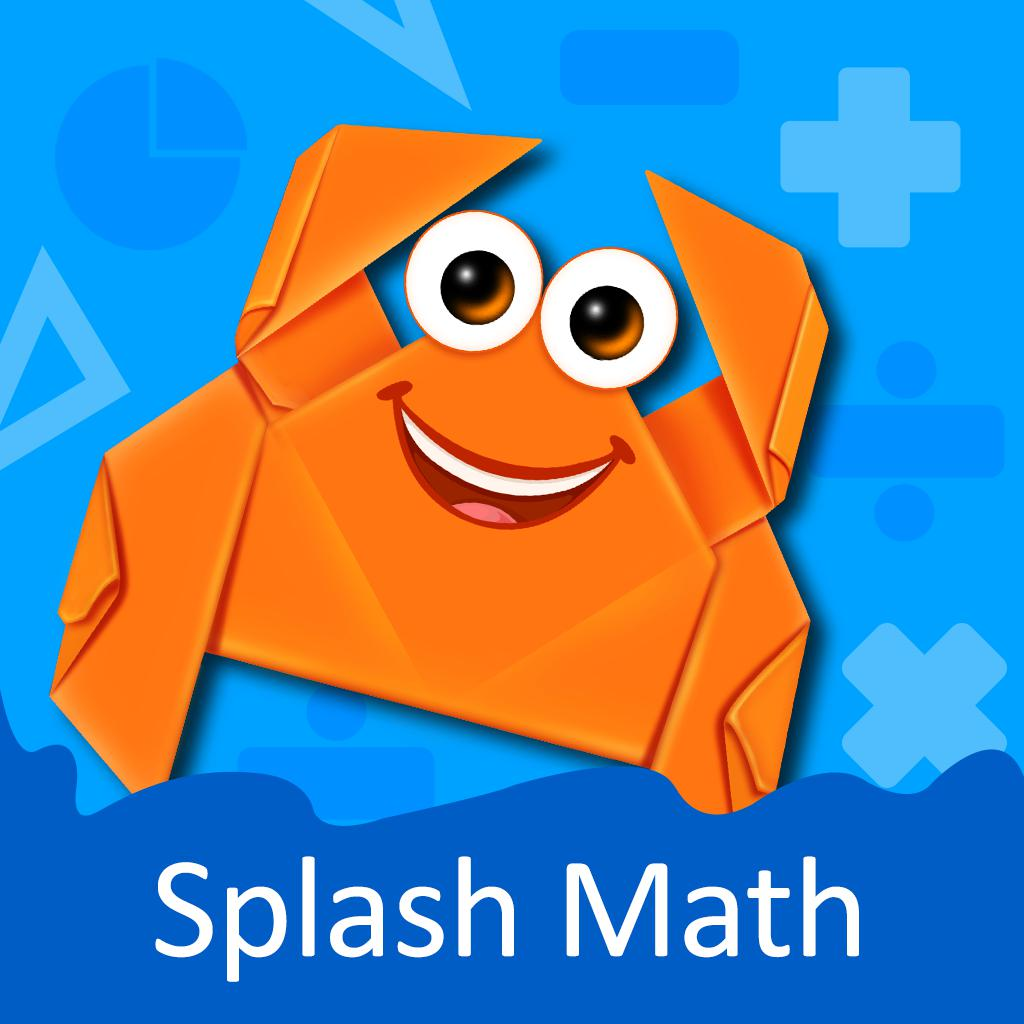3rd Grade Math Games for Kids-Cool app for 7 to 9 year olds 4 4991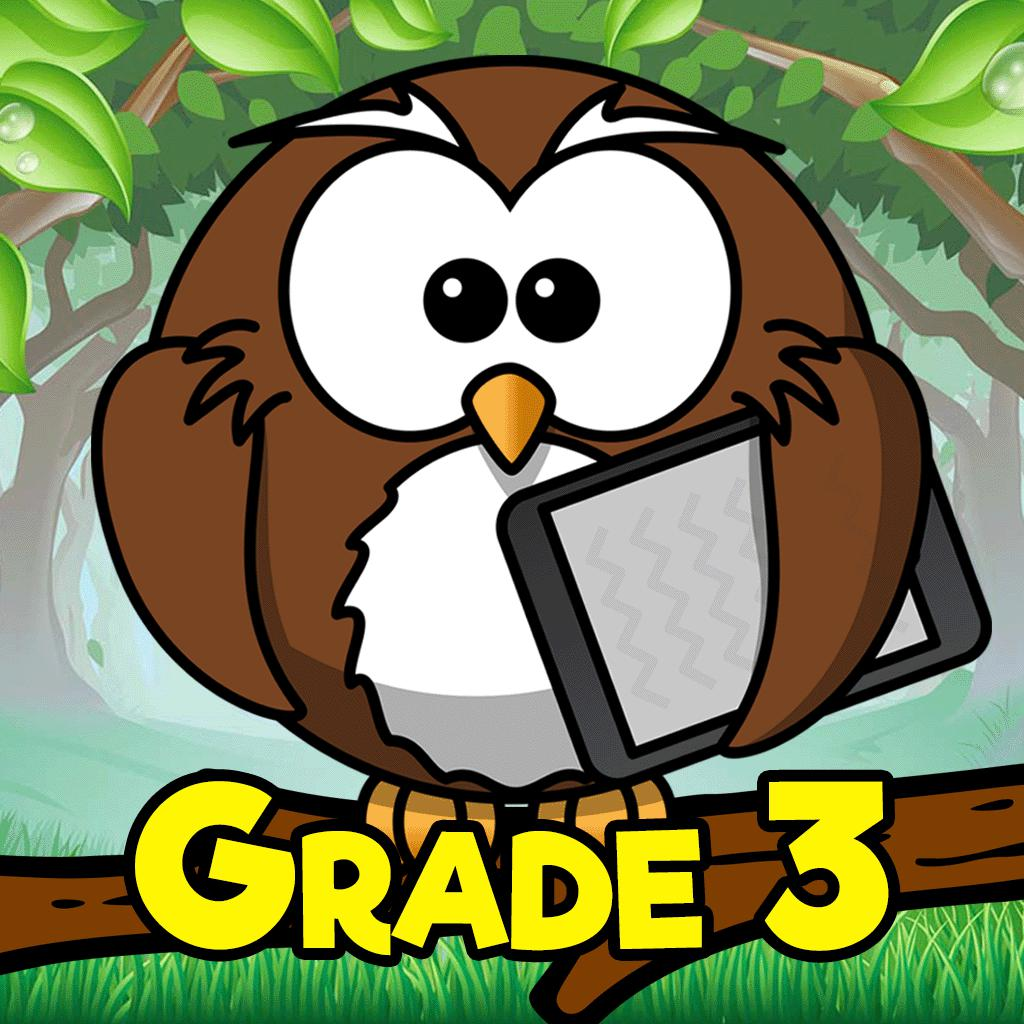Third Grade Learning Games-3rd Grade Math & Reading App 4 7781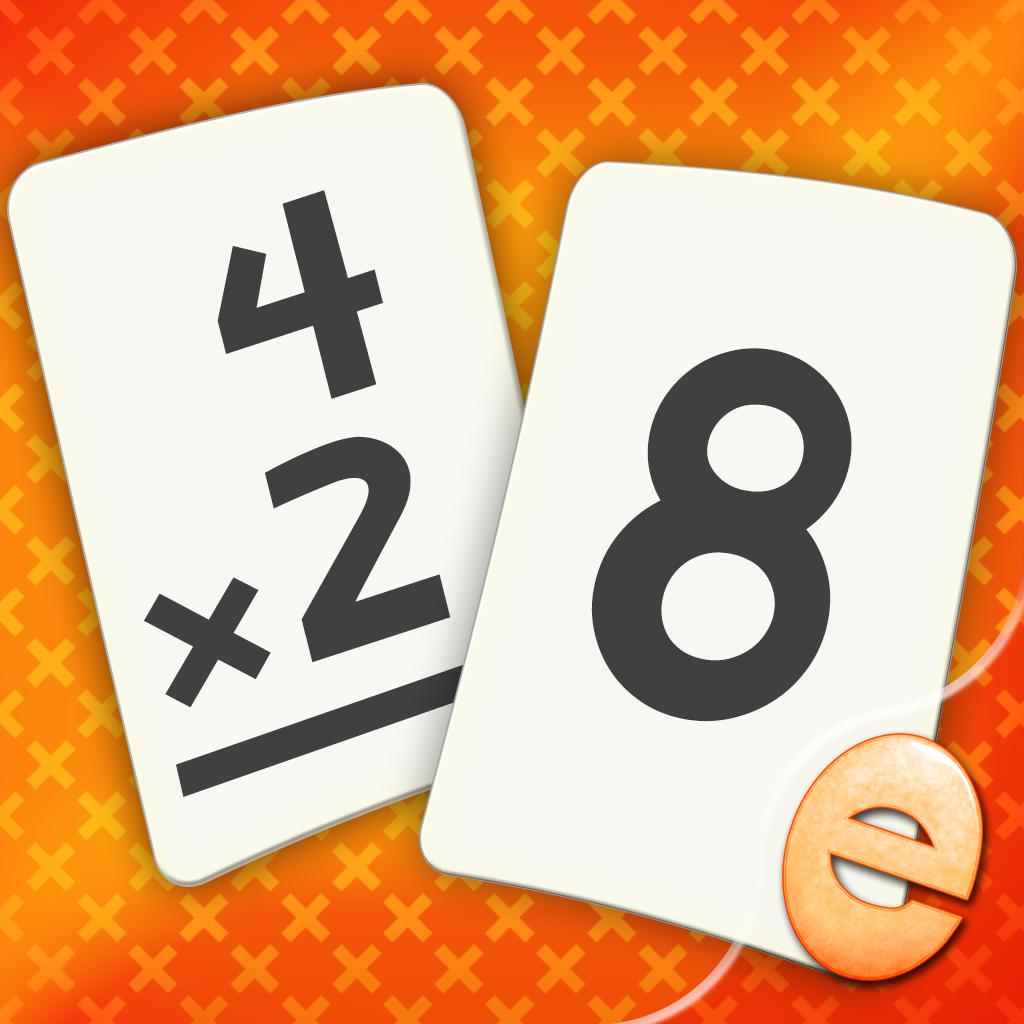Multiplication Math Flashcards-Fun Math Games Practice Drills 4 8713rd Grade Math: Fun Kids Games-Zapzapmath Home - Play & Learn 4 444

201 apps are ranking for "3rd grade math games - multiplication and division" in app store. Top 5 apps are 3rd Grade Math Games - multiplication and division, 3rd Grade Math Games for Kids, Third Grade Learning Games, Multiplication Math Flashcards, and 3rd Grade Math: Fun Kids Games.

As you can see, 3rd Grade Math Games - multiplication and division, 3rd Grade Math Games - multiplication and division, and 3rd Grade Math multiplication and division learning for kids are ranking for multiplication and division games for 3rd graders, also, 3rd Grade Math Games - multiplication and division, 3rd Grade Math Games - multiplication and division, Division and multiplication math games homeschool and Memory Math: +, -, x, / are all competing the keyword division and multiplication math games homeschool.

Before promoting your apps in app store, you'd better perform app analytics for better ASO tactics, and ASOTools, free ASO tools will help you with App store keywords optimization.